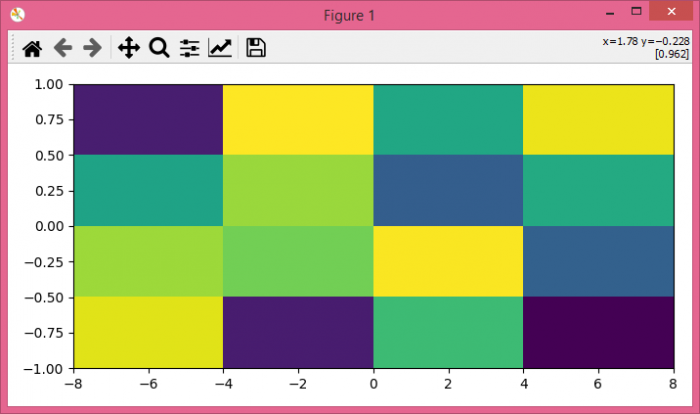# How to change the scale of imshow in matplotlib without stretching the image?

MatplotlibPythonData Visualization

To change the scale of imshow in matplotlib without stretching the image, we can take the following steps.

## Steps

• Set the figure size and adjust the padding between and around the subplots.

• Create random data points with 4×4 dimension.

• Display the data as an image, i.e., on a 2D regular raster.

• Use the extent parameter of imshow to map the image buffer pixel coordinates to a data space coordinate system.

• Next, set the aspect ratio of the image manually by supplying a value such as "aspect=4" or let it auto-scale by using aspect='auto'. This will prevent stretching of the image. By default, imshow sets the aspect of the plot to 1.

• To display the figure, use Show() method.

## Example

import numpy as np
from matplotlib import pyplot as plt

plt.rcParams["figure.figsize"] = [7.00, 3.50]
plt.rcParams["figure.autolayout"] = True

data = np.random.rand(4, 4)

plt.imshow(data, origin='lower', extent=[-4, 4, -1, 1], aspect=4)

plt.show()

## Output

It will produce the following output −